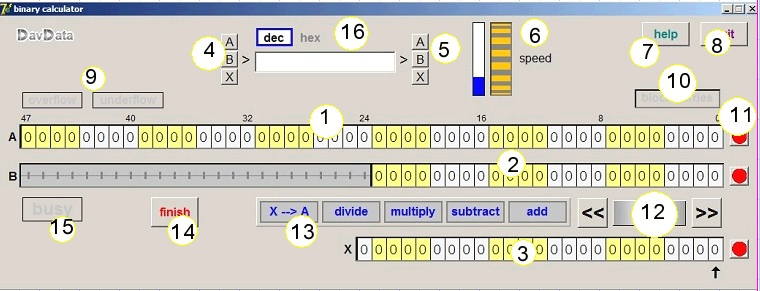Bit operations exerciserdownload excersiser (zip format).

Below is a reduced image of the binary calculator:The picture shows:

 1 A register (bits 0..47) 2 B register (bits 0..23) 3 X register (bits 0..24) 4 A,B,X buttons to display register in decimal or hexadecimal 5 A,B,X buttons to set register bits to value 6 Speed button sets speed from 1 to 25 bit operations per second 7 button to open this page for help 8 button to close this exerciser program 9 overflow and underflow indicators 10 selection button for carry suppression 11 A,B,X clear buttons (red dots) 12 buttons for setting B register position 13 operation select buttons (add,subtract,multiply,divide,X-->A) 14 button to finish operation in progress 15 operation busy indicator 16 edit field showing numbers in decimal or hexadecimal format

Entering a number in a register

binary
Click on bit to set cursor.
Type "0" or "1" to set bit value at cursor position.
Move cursor by pressing > or < cursor keys.

decimal
Select decimal (16)
Type decimal value in editbox and press destination register (5)
Select hex (16)..etc.

Operations with carries

This is the normal way of calculation.
(carry block button -10- is off)

Register B is added to A.
Pressing buttons (12) shifts B realice to register A.
If B is at position n , B is multiplied by 2n).
If the sum exceeds 248-1 (the maximum A register value), the overflow indicator lights.
A mouseclick on the indicator clears it.

SUBTRACT
Register B is added to A.
If B is at position n (B*2n) then the addition sets bit n of register X.
If the difference is negative, the underflow indicator sets.
Clear this indicator by a mouseclick.

MULTIPLY
B is multiplied by X and the product appears in A.
If the product exceeeds the A register, the overflow indicator will light.

DIVIDE
A is divided by B, the quotient appears in X, A holds the remainder.
If the quotient exceeds the X register, the overflowindicator will light.

Operations with carries blocked

Click on button/indicator (10) to set/clear carry blocking.
These operations take place when generating Cyclic Redundancy Codes (CRC).
Addition and subtraction are equal and replaced by Exclusive Or (xor) operations.

Speed control

This bit calculator serves educational purposes.
Therefore, calculations are performed bit by bit, with the speed adjustable from 1 to 25 bits per second.
However, pressing the finish button (14), skips all delays.

Carry and borrow propagation

A left pointing arrow above a bit of register A indicates an incoming carry from a lower bit.
A right pointing arrow indicates that this bit borrowed to the lower bit to make subtraction possible.
A carry generated by A register bit 47 causes overflow to light.
A borrow requested from A register bit 48 causes underflow to light.
A mouseclick on the indicator clears this condition.

If in suppressed carry mode, a divide error causes both overflow and undeflow indicators to light.

The Delphi project

The bit calculator was programmed in Delphi-7.
Some components of my own are used:
- rotation button to set execution speed
- microseconds timer to obtain delays
- arraybuttons to select the type of operation

All code is available here:download Delphi projectdownload Rotation button componentdownload arraybutton and timer components